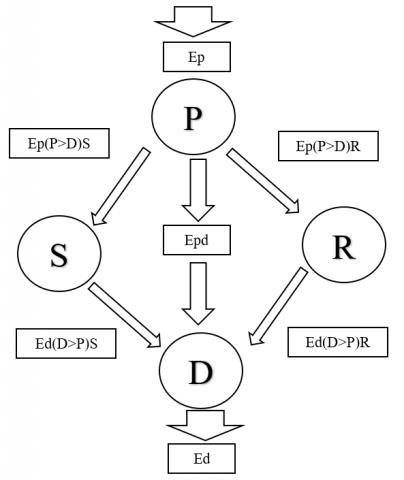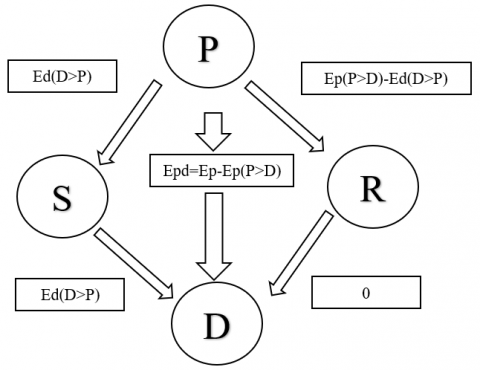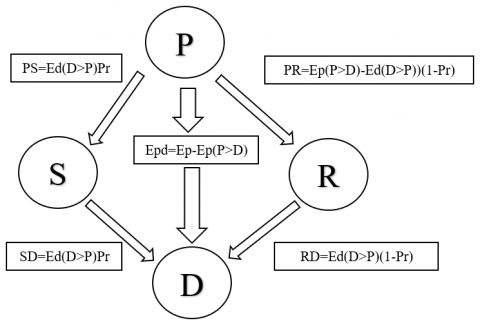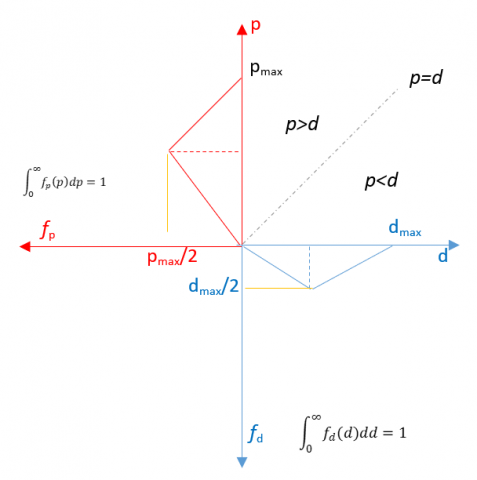# Definition of Probability That Energy Production Differs from Demand, a Statistical Approach

Definition of Probability That Energy Production Differs from Demand, a Statistical Approach
Engineering Department, University of Perugia, Via G. Duranti 93, Perugia 06125, Italy

Universital Mercatorum, Piazza Mattei 10, Rome 00186, Italy

Corresponding Author Email:
Federico.rossi@unipg.it
Page:
236-241
|
DOI:
https://doi.org/10.18280/ti-ijes.652-415
28 March 2021
|
Accepted:
14 May 2021
|
Published:
30 July 2021
| Citation

OPEN ACCESS

Abstract:

Starting from the average trend of energy demand and energy production related to a specific user, the goal is to produce an immediate estimate, as close as possible to the true value (clearly achievable only through a precise and punctual measurement), of the difference existing between these two values, in order to “a priori” understand whether an energy production surplus should be expected, or the opposite trend will occur. If energy produced exceeds the request, two solutions will be possible. An accumulation system can be provided, that allows to avoid taking energy from the grid whenever the trend reserves and the demand exceeds the production. The second solution consists in directly introduce the whole surplus of energy produced in the electricity grid. Similarly, even if the energy required exceeds the amount of energy produced, two different solutions can be envisaged. In both cases, it will be necessary to take energy from the electricity grid. An accumulation system could be created, sized on the maximum difference between production and energy demand, evaluated when the production exceeds the request (if the production never exceeds the request, it would not make sense to talk about accumulation) or, even in this case, a direct exchange might be promoted, both incoming and outgoing, with the electricity grid. Topic of the present paper is to not reasoning any more in terms of energy performances as a function of time but, on the contrary, determine the probability that the difference existing between production and demand assumes a certain value and, based on this, estimate the amount of energy to be stored and/or exchange with the grid.

Keywords:

energy storage, size of accumulation systems, statistical approach

1. Introduction

The term sustainable development means pursuing the needs of current generation and, at the same time, ensuring the maintenance of resources for the next generation . The contemporary history of sustainable development originated with the Brundtland report in 1987, where the World Commission on Environment and Development (WCED) asserted that challenges as the increasing energy consumption, population, and the excessive exploitation of natural resources, represent the most significant obstacles to the sustainable development . Globalization processes characterized the contemporary history of humans and led to a rapid increase and diversification of production and consumption in developing countries. The report well highlighted how, by itself, the economic growth is not enough and the use of less energy intensive applications and the consequent lowering of exploitation of natural sources, is mandatory for sustainability. The use of natural sources as fossil fuels, causes environmental degradation and is also dangerous for human health. That reason encouraged governments to incentivize the diffusion and usage of renewable energy source, as solar and wind, which are widely available in nature and do not damage the environment and the human health.

According to the current estimations, the world population will reach 8.5 billion by 2030 and its too rapid growth will represent a very affective obstacle for the sustainable development . Our capability to reach the 17 sustainable development goals (SDGs) will be decisive to stop the changes caused by our activities. In particular, the 12th, 13th, 14th and 15th SDGs are focused on the sustainable consumption of natural resources and the prevention of damages to the environment associated to deforestation, water pollution, air pollution, desertification and global warming [4, 5]. The main responsible for global warming is carbon dioxide emission in the atmosphere, which reached approximately 34.169 million tonnes in 2019 . However, the concentration of further greenhouse gases has increased in the last years. More in depth, the human activity increased to 405.5 parts per million (ppm) the concentration of carbon dioxide in the atmosphere; methane reached 1859 parts per billion (ppb), while nitrogen dioxide reached 329.9 ppb in 2017. Values as soon shown, means an increase of the respective concentrations of these gases about 146%, 257% and 122%, if compared with the pre-industrial period .

Because fossil fuels utilization represents the main contributor to global warming, the world’s attention in now focused on the possibility to replace them with clean and renewable energy sources . To reduce the global warming, the scientific research plays a key role in making renewable energy sources economically competitive and technically feasible. Despite air and water pollution, added to problems for human health, renewable sources would help in reducing the dependence on fossil fuels, thus increasing the energy security and also counteracting geo-political instability related to the energy sector . Moreover, renewables can help in reducing the energy costs, create new jobs and occupation and so on . In particular, costs associated with energy imports can be drastically reduced through the use of domestic energy productions systems based on renewable energy sources, such as solar, wind, hydropower, biomass, geothermal and so on . By 2025, renewable energy is expected to surpass coal in the production of electricity, while, by 2050, it might represent approximately 50% of the global electricity production .

In this regard, more efforts are required to enhance a widespread utilization of renewable energy sources on a domestic scale. In particular, the energy storage is a crucial challenge in this sense. Despite historical problems, related to renewable energy sources, such as control of transients, quality of energy produced, and so on, also costs associated to purchase, installation and maintenance of energy storage systems, represents a limiting factor in FER diffusion in micro-scale applications. For that reason, the current research on this subject should be particularly involved in optimizing the size of storage devices in function of each specific application, with the aim of reducing costs and size related to the system, while keeping unchanged the storage efficiency.

The present work analyses such problem under the energetical and statistical point of view, providing a detailed description of energy production and energy demand and describing all possible relations which may occur between these two parameters in case of a micro-scale FER-based system. In future researches, this preliminary study will be then deepened with cost analyses, in order to provide a complete model, able to identify, with high accuracy, the optimal size of an energy storage system, in function of the specific application.

2. Model

In this work, the theoretical model proposed in Figure 1 will be discussed in depth.

## 1.pngFigure 1. Possible relations between energy produced and required, in presence of an energy storage system and with the possibility to communicate with the electric grid

In the figure, the energy produced was indicated with “P”, while the energy demand with “D”; letters “S” and “R” indicate respectively the storage system and the electric grid. In addition, the following definitions were used:

• Ep = energy produced;

• Ed = energy required from the users;

• EpS = Energy produced and stored;

• EdS = energy withdrawn from the storage system;

• EpR = energy produced and sent to the grid;

• EdR = energy demand covered from the grid;

• Epd = energy produced and directly consumed.

• Ep(P>D): the sum of instant energy production that exceeds the instant energy demand;

• Ed(D>P): the sum of instant energy demand that exceeds the instant energy production;

The following assumption were made:

Ep(P>D) is equal to the sum of energy stored and energy sent to the grid:

Ep(P>D)=EpS+EpR=Ep(P>D)S+Ep(P>D)R              (1)

At the same time, Ed(D>P) will be equal to the energy taken from the storage system and from the grid:

Ed(D>P)=EdS+EdR=Ed(D>P)S+Ed(D>P)R            (2)

Consequently, the following definitions are true:

Ep=Epd+Ep(P>D)=Epd+Ep(P>D)S+Ep(P>D)R                  (3)

Ed=Epd+Ed(D>P)=Epd+Ed(D>P)S+Ed(D>P)R           (4)

Epd=Ep-Ep(P>D)=Ed-Ed(D>P)        (5)

The terms Ep(P>D) not mean, in absolute terms, that the production is higher than the demand, it simply represents situations during which such relation occurs and indicate the energy production during these time periods. Conversely, the term Ed(D>P) represents energy demand which exceeds the energy produced. In the following discussion, the two opposite possibilities will be analysed:

Ep(P>D)>Ed(D>P)               (6)

Ed(D>P)>Ep(P>D)              (7)

3. Discussion

In this section, the two possible relations between Ep(P>D) and Ed(D>P) will be analysed. In both cases, a scheme similar to that of Figure 1, will be used to provide a fast comparison.

3.1 Ep(P>D) > Ed(D>P)

The present case describes situation where the overall amount of energy produced, during a specified time period, is greater than the demand of energy registered during the same period. The energy demand will be mainly covered directly by energy production; however, short periods, during which the demand exceeds the production, may occur. Thus, the necessity to consider the use of an energy storage system and to communicate with the grid.

The energy produced consist of the sum of energy produced and immediately consumed Epd and Ep(P>D). This latter quantity may be stored or send to the grid and both contributions must be considered:

Ep =Epd+Ep(P>D)=Epd+Ep(P>D)S+Ep(P>D)R               (8)

The demand of energy can be written as the sum of energy produced and immediately consumed and the quantity of energy required, which cannot be immediately satisfied from the energy production system. This amount may be obtained from the storage system or from the grid:

Ed=Epd+Ed(D>P)S+Ed(D>P)R                      (9)

Because of the energy produced is greater than the energy demand, when this latter parameter exceeds the current production, it might be entirely satisfied with energy stored. The energy produced will cover the direct consumption and is enough to cover the indirect consumption. If these two quantities are covered, the energy produced in excess is sent to the grid. Consequently, the communication with the grid will consists of a release of energy (from the production system to grid), when it is possible, and anything else. In this case, energy will never be withdrawn from the grid.

According to the previous considerations, the demand in excess will be entirely covered from a portion of the production in excess:

Ed(D>P)S=Ep(P>D)S              (10)

Conversely, energy will never be provided from the grid:

Ed(D>P)R=0                        (11)

The demand of energy, evaluated in time periods during which the demand is greater than the production, is equal to:

Ed(D>P)=Ed(D>P)S+Ed(D>P)R=Ed(P>D)S                  (12)

The amount of energy produced and sent to the grid, obviously coincides with the quantity which was not required from the grid and can be written as follows:

Ep(P>D)R=Ep(P>D) – Ep(P>D)S=Ep (P>D) – Ed(D>P)S=Ep(P>D)–Ed(D>P)           (13)

It consists of the difference between energy produced in excess and energy stored. This second parameter can be written as a function of the energy demand, according to Equation .., and, considering the all energy demand required when the demand exceeds the production, is covered by the energy previously stored, this term is equal to the whole demand in excess.

Figure 2 reports the scheme, previously shown in Figure 1, adapted to the situation as soon detailed.

In conclusion, the size of the accumulation system might be dimensioned by considering parameter Ed(D>P), or the excess of energy demand, clearly evaluated when the demand exceeds the production.

In this preliminary article, economic considerations were not taken into account. Even if energy demand might be entirely satisfied by direct production and energy stored (when the production exceeds the demand), or in situations that can be reconnected to what describe above, considerations about costs may allow to different practical variation.

## 2.pngFigure 2. Possible relations between energy produced and required, when the overall energy production is greater than the energy demand, which can be completely satisfied with energy stored

For instance, the most competitive solution might consist of do not satisfy completely the energy demand with energy directly produced and stored (even when it is possible): associated to current prices and their variations during the daytime, it may be cheaper to send a greater portion of the overproduction of energy to the grid and satisfy a part of the demand by withdrawing energy from the grid.

The scheme, previously described in Figure 2, would appear as in the following Figure 3:

## 3.pngFigure 3. Possible relations between energy produced and required, when part of the demand is satisfied with energy coming from the grid, even if the overproduction of energy may be enough to entirely satisfy it

The term “Pr” is the percentage of Ed(D>P) satisfied with energy previously stored, while (1-Pr) describes the quantity covered with the grid, which, in this case, is not equal to zero. The main advantage of this model stays in the possibility of adapting it to the current energy costs and their variability during the daytime, by simply intervening on parameter Pr.

3.2 Ed(D>P) > Ep(P>D)

This second case describes situations where the overall demand exceeds the overall production in a specific time period. It means that energy demand, measured when the demand is greater than the production, is higher than the energy production, measured when the production is greater than the demand.

Consequently, even if the whole overproduction of energy is stored, it will not be enough to satisfy the whole energy demand and part of energy required will be necessarily withdrawn from the grid (see Figure 4).

## 4.pngFigure 4. Possible relations between energy produced and required, when the overall energy demand is greater than the energy production and part of energy required will be necessarily provided from the grid

In this case, the size of the storage system can be defined as a function of Ep(P>D), or as a function of the maximum energy which can be stored.

The demand of energy will benefit of three different contribution:

1. Epd: energy produced and directly consumed;

2. Ep(P>D)S: energy stored when the production exceeds the demand

3. Energy coming from the grid, which will be used whenever the other two contributions will be not sufficient.

## 5.pngFigure 5. Possible relations between energy produced and required, when part of the demand is satisfied with energy coming from the grid since overproduction of energy is not enough to entirely satisfy it

Also in the present case, considerations based on energy costs could make, the possibility of sending part of the energy produced to the grid, preferable. The same parameter Pr introduced in the previous section, allows to integrate such consideration in the model, thus leading to the scheme shown in Figure 5.

3.3 Demand and production are random: continuous model

In Figure 6 a 2-dimensional graph of the random variables “d” and “p” is sketched. Let’s specify that maximum values od “d” and “p” generally correspond to maximum limit of energy supply guaranteed to the customer and nominal power of non-programmable energy source.

## 6.pngFigure 6. Both demand and production are random and represented by triangular distributions. The line d=p is the loci where demand equalizes production

As it may be seen, the space below the line p=d represents the region where demand exceeds the production and the space above the line p=d represents the region where production exceeds demand.

Conditional probabilities are used to assess the probability that demand overtakes production P(d>p):

$P(d>p)=\int_{0}^{d_{\max }} \int_{0}^{d} f_{p}(p) \cdot f_{d}(d) \cdot d p \cdot d d$                     (14)

By the other hand, probability that production equalizes or overtakes demand $\mathrm{P}(p \geq d)$ is:

$P(p \geq d)=\int_{0}^{p_{\max }} \int_{d}^{p_{\max }} f_{d}(d) \cdot f_{p}(p) \cdot d p \cdot d d$          (15)

Thus, the statistical difference between demanded energy and produced one, may be calculated by the following relation:

$E(d>p)=T \int_{0}^{d_{\max }} \int_{0}^{d} f_{p}(p) \cdot f_{d}(d) \cdot(d-p) \cdot d p \cdot d d$                 (16)

Eq. (16) represents the mean energy required by the demand which is not supplied by the production.

Thus, also for this case, the storage size is set as S=E(d>p).

The mean produced energy surplus may be also calculated via Eq. (17):

$E(p \geq d)=T \int_{0}^{p_{\max }} \int_{d}^{p_{\max }} f_{d}(d) \cdot f_{p}(p) \cdot(p-d) \cdot d p \cdot d d$                     (17)

Thus S=E(d>p) may be obtained by using a share of E(p>d).

Considerations concerning Eq. 9 are also valid in this case.

Hoverer, on behalf pf probability density, only discrete normalized frequency of demanded and produced power may be retrieved from real data; let’s call them respectively fdi and fpj. Each ith and jth frequency is related to the discretized demanded power di and produced power pj. The demanded power is discretized in imax intervals and the produced power in jmax intervals. The width of each interval is then calculated as:

$\Delta d=\frac{T}{i_{m a x}}$ and $\Delta p=\frac{T}{i_{\max }}$                         (18)

Thus, neglecting prodromal considerations, Eqns. (14), (15), (16) and (17) may be rewritten in terms of normalized discrete frequencies and they respectively become:

$P(p>d)=\sum_{i=1}^{i_{\max }} \sum_{i=1}^{i-1} f d_{i} \cdot f p_{i}$                 (19)

$P(p \geq d)=\sum_{i=1}^{i_{\max }} \sum_{j=i}^{j_{\max }} f d_{i} \cdot f p_{j}$            (20)

$E(d>p)=T \cdot \sum_{i=1}^{i_{\max }} \sum_{i=1}^{i-1} f d_{i} \cdot f p_{i} \cdot\left(d_{i}-p_{i}\right)$              (21)

$E(d \geq p)=T \cdot \sum_{i=1}^{i_{\max }} \sum_{j=i}^{j \max } f d_{i} \cdot f p_{j} \cdot\left(p_{j}-d_{i}\right)$              (22)

Considerations on storage size “S” and energy surplus “F” keep on working also adopting Eqns. (21) and (22) on behalf of e (16) and (17).

4. Discussion

Before discussing about model validation method let’s make some interesting examples where demand and production probability distribution are uniform between 0 and respectively dmax and pmax; thus uniform probability densities are respectively: “1/dmax and “1/pmax”.

In this case:

$P(p>d)=\int_{0}^{d_{\max }} \int_{0}^{d} \frac{1}{d_{\max }} \cdot \frac{1}{p_{\max }} \cdot d p \cdot d d=\frac{d_{\max }}{2 \cdot p_{\max }}$                                (23)

$P(p \geq d)=\int_{0}^{d_{\max }} \int_{\mathrm{d}}^{p_{\max }} \frac{1}{d_{\max }} \cdot \frac{1}{p_{\max }} \cdot d p \cdot d d=1-\frac{d_{\max }}{2 \cdot p_{\max }}$                 (24)

And:

$E(d>p)=T \int_{0}^{d_{\max }} \int_{0}^{d} \frac{1}{d_{\max }} \cdot \frac{1}{p_{\max }} \cdot(d-p) \cdot d p \cdot d d=T \cdot \frac{d_{\max }^{2}}{6 \cdot p_{\max }}$           (25)

$E(d \geq p)=T \int_{0}^{d_{\max }} \int_{d}^{p_{\max }} \frac{1}{d_{\max }} \cdot \frac{1}{p_{\max }} \cdot(p-d) \cdot d p \cdot d d=T \cdot\left(\frac{p_{\max }}{2}-\frac{d_{\max }}{2}+\frac{d_{\max }^{2}}{6 \cdot p_{m a x}}\right)$            (26)

For this case, the optimal storage size is set as $\mathrm{S}=T \cdot \frac{d_{\max }^{2}}{6 \cdot p_{\max }}$ and the average amount of emery which may be dispatched away (to the grid) becomes $\mathrm{F}=T \cdot\left(\frac{p_{\max }}{2}-\frac{d_{\max }}{2}\right)$.

Although the previous optimization was done for uniform energy distribution, they are supposed to work also for the most of real cases; in fact, periodical energy demand and energy productions (hourly variation) have roughly uniform probability distribution. Thus Eq. (25) may be considered as a “at glance criterion” for setting the storage size. Anyway, in case of more random energy distributions, Eqns. (25) and (26) may be calculated using proper and specific probability densities of demand and produced energy as well as triangular, Raleigh and quasi-gaussian (summation of a finite set of uniform densities).

5. Conclusions

The increasing demand of electrical energy, together with the current transition of the energy sector towards a scenario dominated from the renewable energy sources, requires a progressive abandonment of fossil fuels and the optimization of energy storage systems, which allow to drastically contain challenges due to the non-programmability of these energy sources.

For each probability density function, associated to the production or to the demand of energy, an optimal quantity of energy to store can be defined, through a stochastic model. Renewable energy sources, due to their non-programmability, can be described with random-type production values. The stochastic model proposed in this work, aims to define with high accuracy the relation existing between the random-type production with the function associated to the energy demand, in order to define an optimal size of the storage device, for each specific energy production system and, in particular, for small-scale applications. Future works will be focused on improving such model by introducing also variables concerning economic aspects, which will be useful to establish the optimal size in terms of costs.

References

 Gyamfi, S., Derkyi, N.S., Asuamah, E.Y., Aduako, I.J. (2018). Renewable energy and sustainable development. In Sustainable Hydropower in West Africa, 75-94. Academic Press. https://doi.org/10.1016/B978-0-12-813016-2.00006-X.

 Pata, U.K. (2021). Linking renewable energy, globalization, agriculture, CO2 emissions and ecological footprint in BRIC countries: A sustainability perspective. Renewable Energy, 173: 197-208. https://doi.org/10.1016/j.renene.2021.03.125

 United Nations, World population prospects: the 2019 version. https://population.un.org/wpp/Publications/Files/WPP2019_Highlights.pdf.

 United Nations, Transforming our world: the 2030 agenda for sustainable development. https://sustainabledevelopment.un.org/content/documents/21252030%20Agenda%20for%20Sustainable%20Development%20web.pdf,2015.

 IPCC Working Group III, Climate change 2014-mitigation of climate change: synthesis report, Intergovernmental Panel on Climate Change Cambridge University Press, Cambridge, United Kingdom and New York, NY, USA. (2014).

 BP, Statistical review of world energy. https://www.bp.com/content/dam/bp/business-sites/en/global/corporate/xlsx/energy-economics/statistical-review/bp-stats-review-2020-all-data.xlsx, 2020.

 World Meteorological Organization, WMO statement on the state of the global climate in 2018.https://library.wmo.int/doc_num.php?explnum_id¼5789,2019.

 Elum, Z.A., Momodu, A.S. (2017). Climate change mitigation and renewable energy for sustainable development in Nigeria: A discourse approach. Renewable and Sustainable Energy Reviews, 76: 72-80. https://doi.org/10.1016/j.rser.2017.03.040.

 Al-Mulali, U., Solarin, S.A., Sheau-Ting, L., Ozturk, I. (2016). Does moving towards renewable energy cause water and land inefficiency? An empirical investigation. Energy Policy, 93: 303-314. https://doi.org/10.1016/j.enpol.2016.03.023.

 REN21, Renewables. Global status report (GSR) Paris: REN21 secretariate. https://www.ren21.net/wp-content/uploads/2019/05/gsr_2020_full_report_en.pdf, 2020.

 Edenhofer, O., Pichs-Madruga, R., Sokona, Y., Seyboth, K., Kadner, S., Zwickel, T., Matschoss, P. (2011). Renewable energy sources and climate change mitigation: Special report of the intergovernmental panel on climate change. Cambridge University Press. https://doi.org/10.1017/CBO9781139151153.

 IA, International energy outlook 2019 with projections to 2050. US Energy Information Administration. https://www.eia.gov/outlooks/ieo/pdf/ieo2019.pdf, 2019.